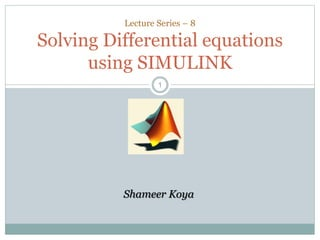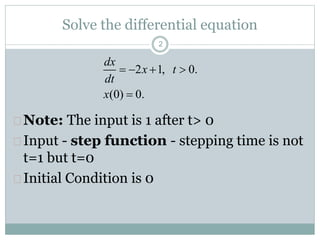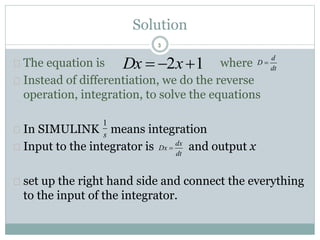Successfully reported this slideshow.

# Mat lab solving equations simulink

3

Share×
1 of 11
1 of 11

# Mat lab solving equations simulink

3

Share

## More Related Content

### Related Books

Free with a 14 day trial from Scribd

See all

### Related Audiobooks

Free with a 14 day trial from Scribd

See all

### Mat lab solving equations simulink

1. 1. 11 Lecture Series – 8 Solving Differential equations using SIMULINK Shameer Koya
2. 2. Solve the differential equation  Note: The input is 1 after t> 0  Input - step function - stepping time is not t=1 but t=0  Initial Condition is 0 2 2 1, 0. (0) 0. dx x t dt x     
3. 3. 3 Solution  The equation is where  Instead of differentiation, we do the reverse operation, integration, to solve the equations  In SIMULINK means integration  Input to the integrator is and output x  set up the right hand side and connect the everything to the input of the integrator. 2 1Dx x   d D dt  1 s dx Dx dt 
4. 4. 4
5. 5.  Another issue that we have not considered is initial condition.  As you know every differential equation should have initial conditions given.  The default value in SIMULINK is zero.  To change that click the integrator. 5 Double-click Initial condition
6. 6.  If you want to see both the input and output at the same time, use Mux (multiplexer) block, which you can find under Signals and Systems block library 6
7. 7. Higher order differential equation  Second order equation  To set up the right-hand side two integrators are needed  The input to the first integrator is the second derivative and its output is  second integrator producing x(t) at its output 7 2 2 5 9 d x dx x dt dt    d x dt 2 2 dx dt (0) 2 (0) 2 dx x dt x     &
8. 8.  Assume that u(t) = 0, that is, there is no input. 8 2 2 5 9 d x dx x dt dt   
9. 9.  Set the initial conditions of the integrator 9
10. 10. Ouput 10
11. 11. 11 Thanks Questions ??